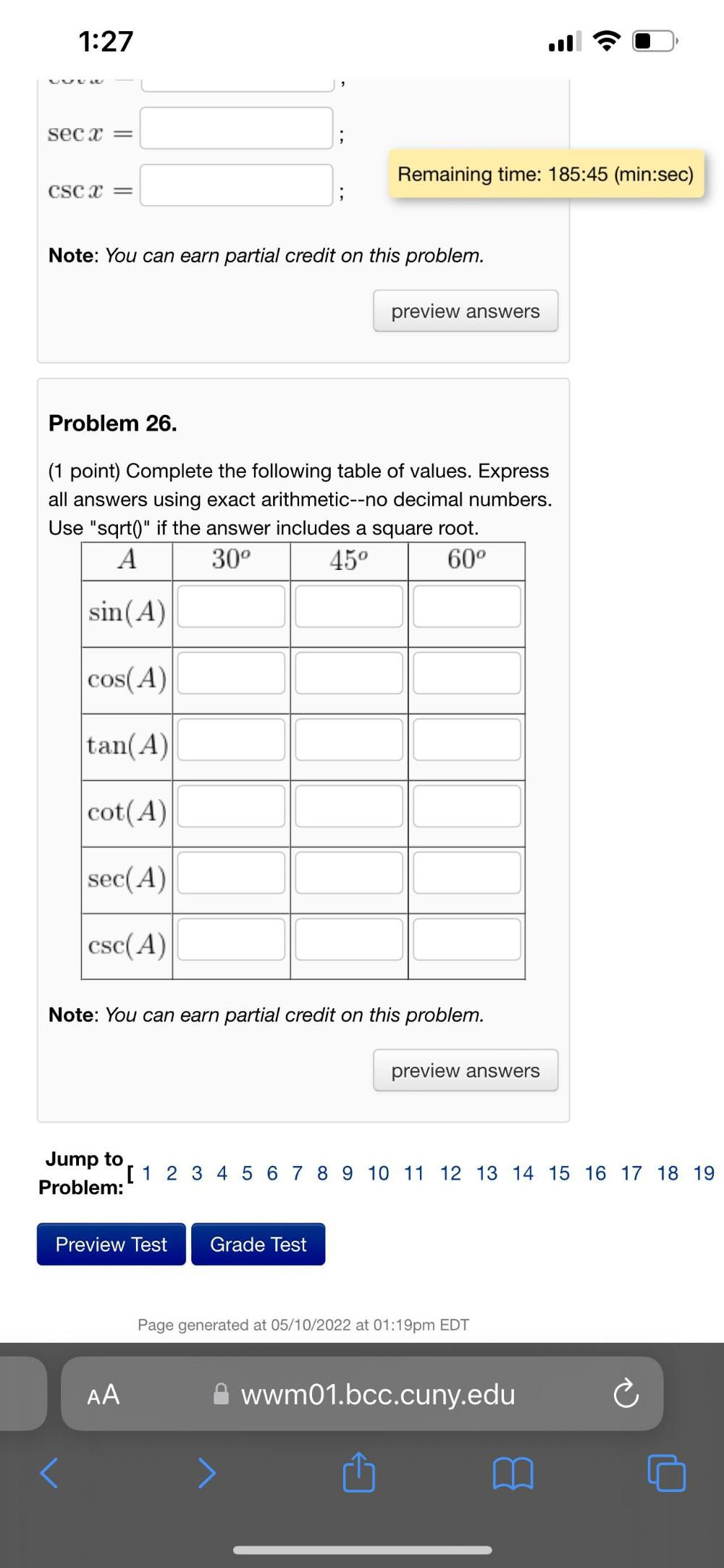Question:

# Complete the following table of values. Express all answers using exact arithmetic--no decimal numbers. Use "sqrt()" if the answer includes a square root. A 30°Complete the following table of values. Express all answers using exact arithmetic--no decimal numbers. Use "sqrt()" if the answer includes a square root. A 30° 45° 60° sin(A) ______ ______ ______ cos(A) ______ ______ ______ tan(A) ______ ______ ______ cot(A) ______ ______ ______ sec(A) ______ ______ ______ csc(A) ______ ______ ______